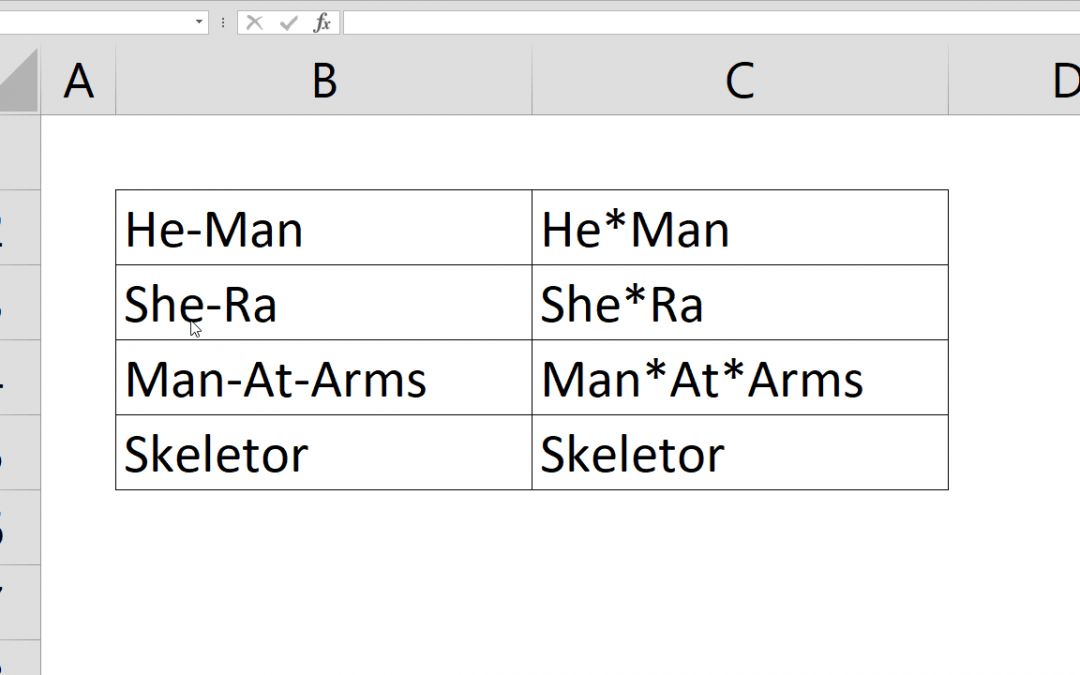## How to replace characters in Excel

Excel’s built in find and replace feature is fairly useful in most cases. But this can get tedious if you’re working with a large workbook or want to set this up dynamically. In this article we’re going to show you how to replace characters in Excel...## How to sum by date in Excel without time

Excel already has functions like the SUMIF and the SUMIFS for summing data by groups. However; they can't work if you have date-time values combined. This article shows How to sum by date in Excel without time using SUMPRODUCT and INT functions. Syntax =SUMPRODUCT(...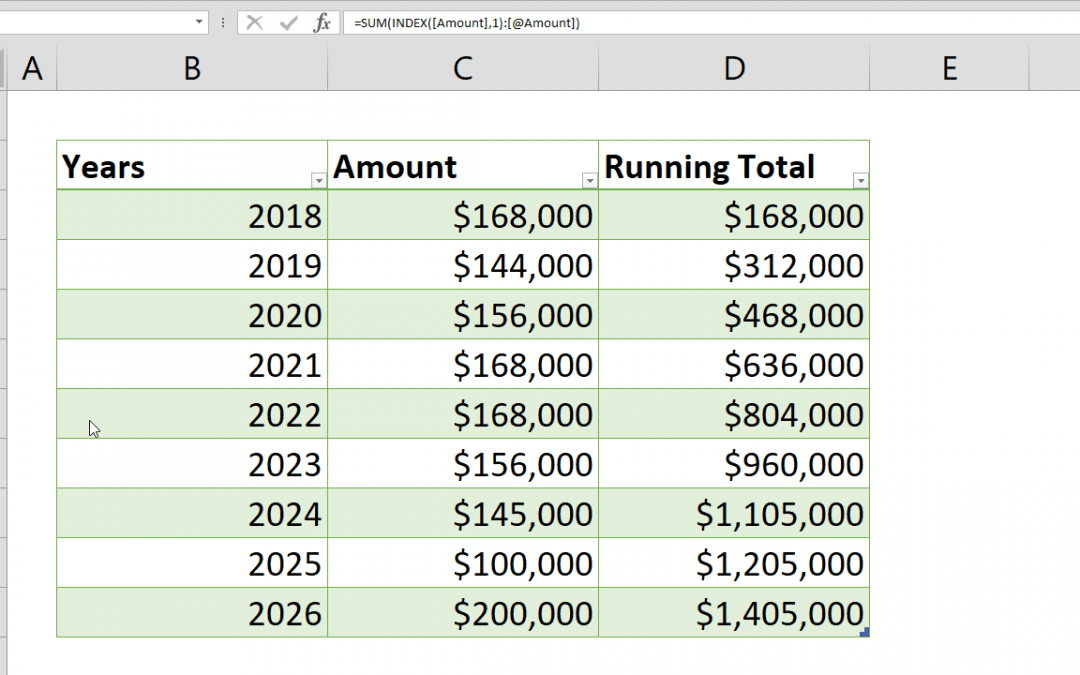## How to calculate running totals in Excel Tables

This article shows how to calculate running totals in Excel Tables by using structured references. If your data is not in a Table, please continue with this article: How to calculate running count Syntax =SUM(INDEX([column of values],1):[@ column of values]) Steps...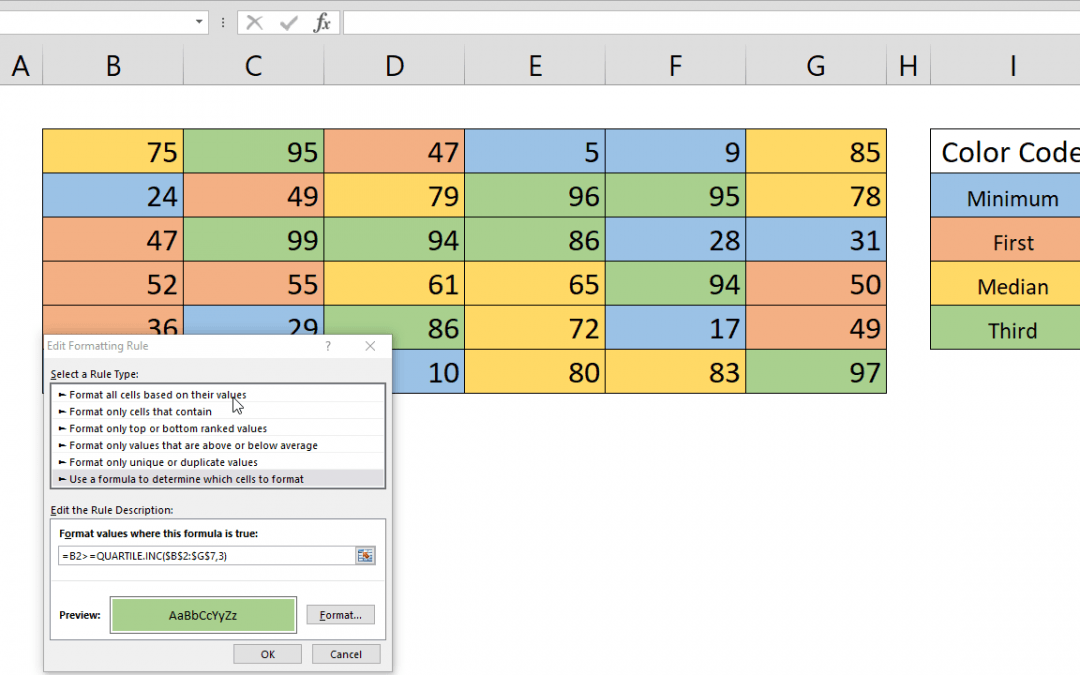## How to highlight quartiles in Excel

It is often a good idea to highlight duplicate values in a data set to help easily identify the outliers. In this article we are going to explain how to find quartiles in Excel and highlight them dynamically with Conditional Formatting. Syntax = relative reference of...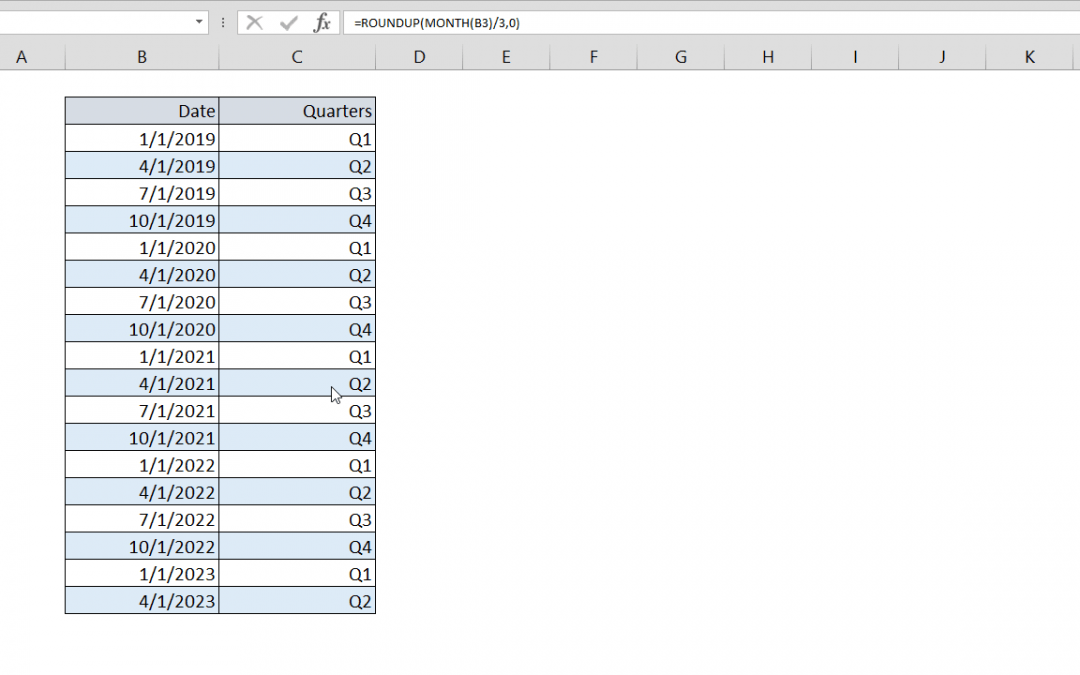## How to create a Quarter formula in Excel

There is no specific Quarter formula in Excel. But an Excel quarter formula can be constructed by combining ROUNDUP and MONTH functions with custom number formatting. Syntax =ROUNDUP( MONTH( date value ) /3, 0 ) Steps Start with =ROUNDUP( function Continue with MONTH(...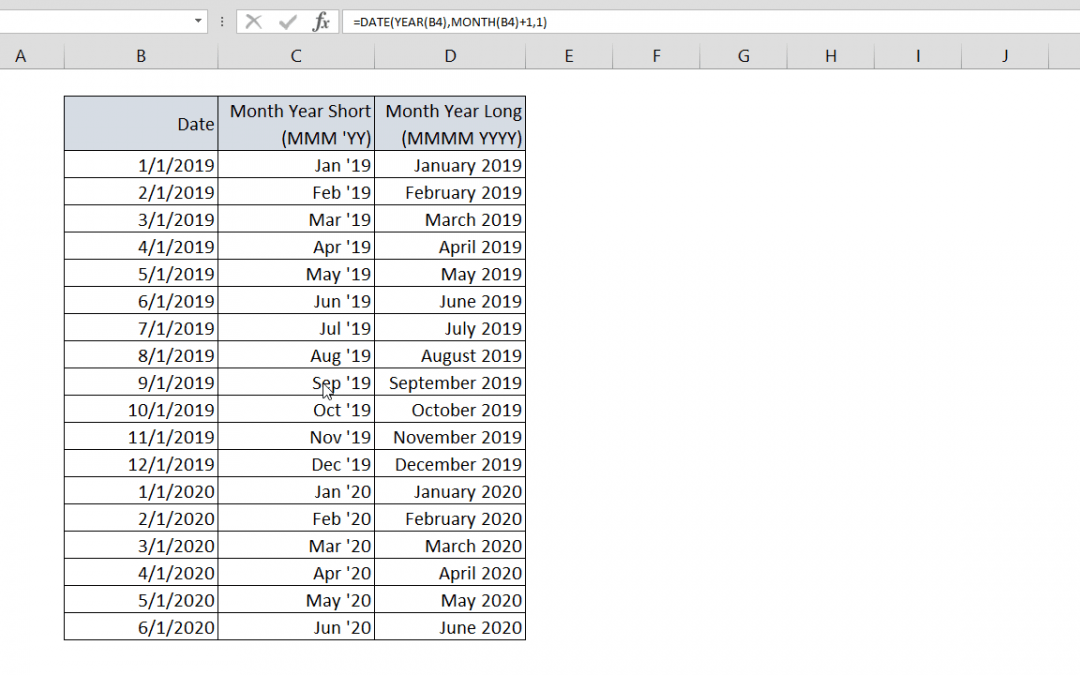## How to generate Excel month names

This article shows you how to generate month list by formulas and how to display Excel month names instead of actual date values. We will use DATE, YEAR and MONTH formulas as well as Number Formatting. Syntax =DATE( YEAR( previous date ), MONTH( previous date )+1, 1)...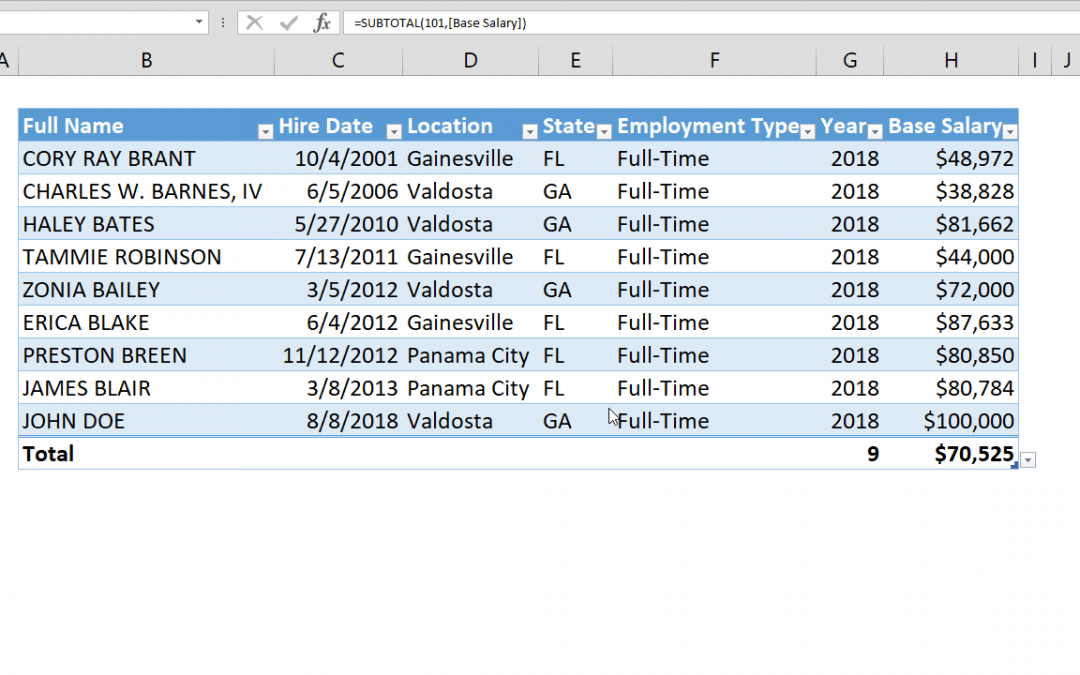## How to calculate Excel Table totals

This article shows how to calculate Excel table totals by using Excel Table which is one of the most underrated features in Excel. Steps Select a cell inside your table or whole range Press Ctrl + T or click Table icon under INSERT tab on the ribbon Make sure My table...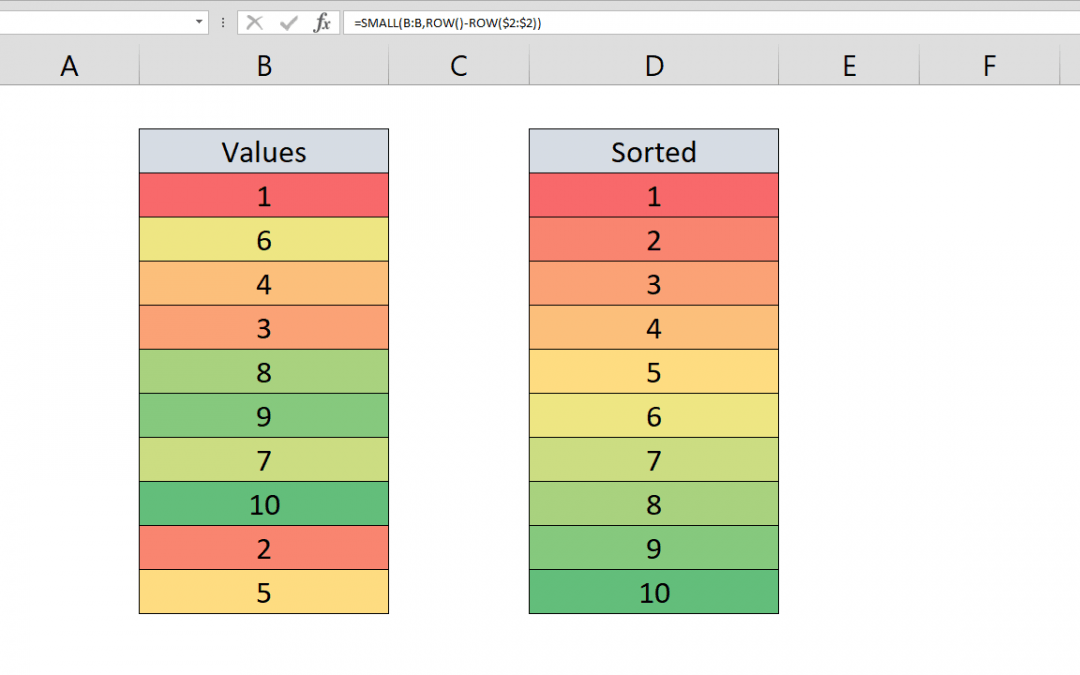## How to use Excel sort function

Although Excel has a built-in sort feature, there are times you may need to sort data by formula or in a formula. This article shows how to use Excel sort function with SMALL and ROW functions. Syntax =SMALL( column of list, ROW()-ROW(row of list’s title)) Steps...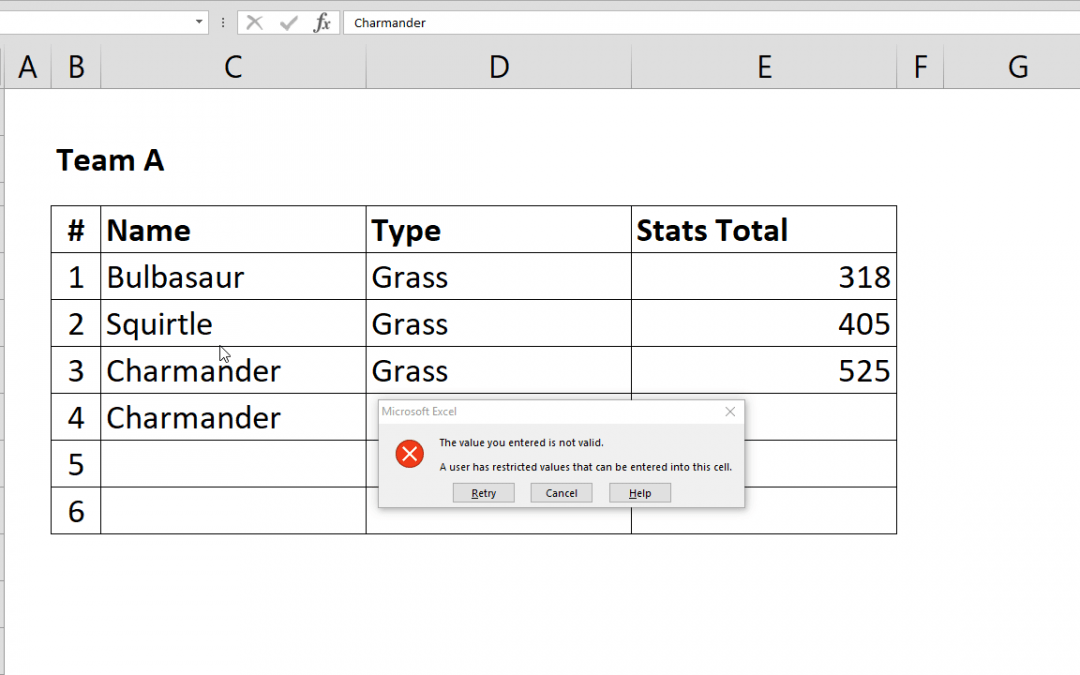## How to create Excel data validation for unique values

Entering data into an Excel file manually carries the risk of incorrect entries. Using Excel data validation feature reduces and in many cases complete eliminates this risk. In this article, we are going to show how to combine COUNTIF function with Data Validation...